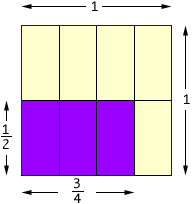Name: Angela Elementary level I'm a teacher. Questions: 1. represent multiplication of fractions via an area model 2. describe why, not just how, to round decimals Thank You! Hi Angela, If your students have calculated the area of a rectangle with integer sides by multiplying the integers then you can use this concept to represent the multiplication of fractions. Suppose that you want to multiply  1/2 and  3/4. Draw a unit square and divide it in half in one direction and in quarters in the other. The region shaded in purple below has an area represented by  1/2 x 3/4In this construction you divided the unit square into eight, equal sized rectangles so each rectangle represents one-eighth on the unit square. Hence the purple region is three-eights of the unit square so that  1/2 x 3/4 = 3/8 For your second question one example that immediately come to mind is with money. Suppose that you purchased something for \$28.95 and you have to pay 7% tax. Then the tax is \$28.95 x 0.07 = \$2.0265. Since we don't have coins worth less than \$0.01 we round the tax to \$2.03. Cheers, Penny Go to Math Central